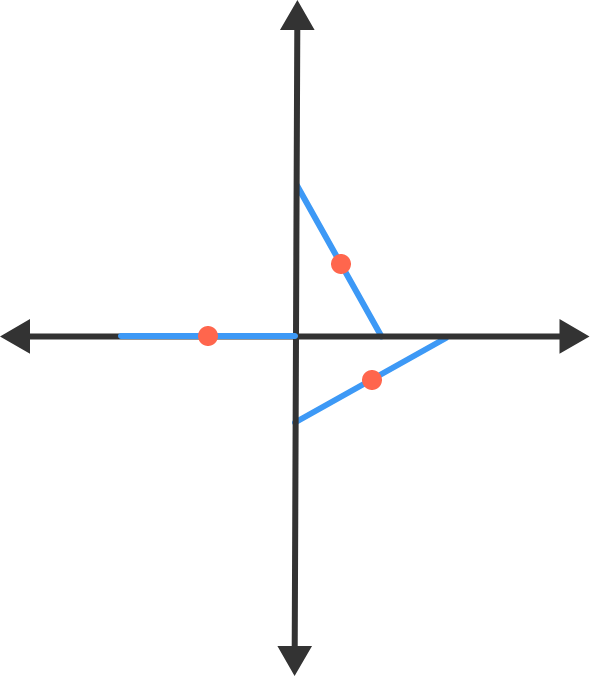# Equation Of LocusA rod of length $l$ slides with its ends on the $x$-axis and $y$-axis.

Find the locus of its midpoint.

×